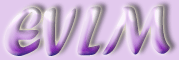Arithmetic operations on integers

Addition is the basic operation of arithmetic and is denoted by the symbol, denoting positive. In its simplest form, addition combines two numbers into a single number which is called the sum e.g..

# 2  Subtraction

Subtraction is the opposite of addition and is denoted by the symbol, denoting negative. Subtraction finds the difference between two numbers. If two numbers are equal, their difference is zero e.g.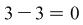. If they are not equal, the sign of the difference is decided by the order of the subtraction. If the first number is larger than the second, the difference is positive e.g., and if the first number is smaller than the second, the difference is negative e.g.. The order of the subtraction can be changed as long as care is taken to position the negative symbol correctly e.g.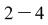can be written as.

# 3  Multiplication

Multiplication is essentially the same as repeated addition. It is generally denoted by the symbol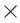although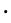and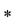are also commonly used. Multiplying a number by 2 is the same as adding the number to itself once e.g.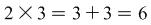. In a similar way, multiplying a number by 3 is the same as adding the number to itself twice e.g.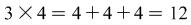.

# 4  Division

Division is essentially the opposite of multiplication, and is denoted by the symbol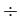. Dividing a number by 2 is the same as multiplying it bye.g.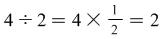.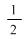is called the reciprocal of 2. In the same way, dividing a number by 3 is the same as multiplying it by e.g. The first number is called the dividend, the second number is called the divisor of the first and the result of the division is called the quotient. In the last example, 6 is the dividend, 3 the divisor and 2 is the quotient.

# 5  Lowest common denominator

The lowest common denominator (LCD) of two integers is the smallest integer which can be divided by both integers.

## 5.1  Example

Find the LCD of 6 and 3.

Solution:

Clearly, 6 can be divided by itself, and 3 divides 6 twice. The LCD of 6 and 3 is therefore 6.

# 6  Highest common factor

The highest common factor (HCF) is the largest integer which is a common divisor of two integers.

## 6.1  Example

Find the HCF of 18 and 12.

Solution:

18 can be divided by 1, 2, 3, 6, 9 and 12 can be divided by 1, 2, 3, 4, 6. 6 is the largest integer which divides both 18 and 12, so is the required HCF.

# 7  Brackets

Brackets are used to group numbers together before further arithmetic operations are carried out.

Examples

1. Evaluate.

Solution:

The calculation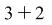is carried out inside the brackets before multiplying the result by 3 i.e.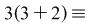(meaning “is equivalent to”)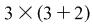. Consequently,.

It is also possible to carry out the same computation using a different method:

Solution:

Each number inside the bracket is multiplied by 3 and the resulting two numbers are added together i.e.